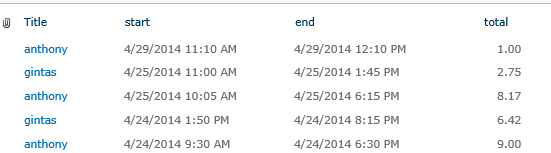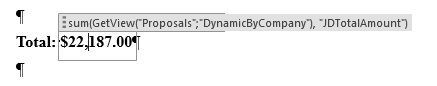Sum function

Applies to JungleDocs for SharePoint 2010-2019.
For JungleDocs for Office 365 tutorials, click here.

This article describes the formula syntax and usage of the Sum function in JungleDocs.

Description

Returns the sum of values from a SharePoint list column.

Syntax

Sum(recordSet; fieldTitle)

The Sum function syntax has the following arguments:

• RecordSet   Required. Any command that returns a list of items (e.g., GetView).
• FieldTitle   Required. The title of the field with values you want to sum.

Example

Let's say you have a TimeSheet list's AllItems view that contains numeric values in the total field:Here are some examples of how you can use the Sum function:

Sum(GetView("TimeSheet"; "AllItems"); "total")

Sums values by the total field.

Sum(ReportItems; "total")

Sums values for selected items by the total field.

You can also sum field values for a particular company using GetView with dynamic view, for example: